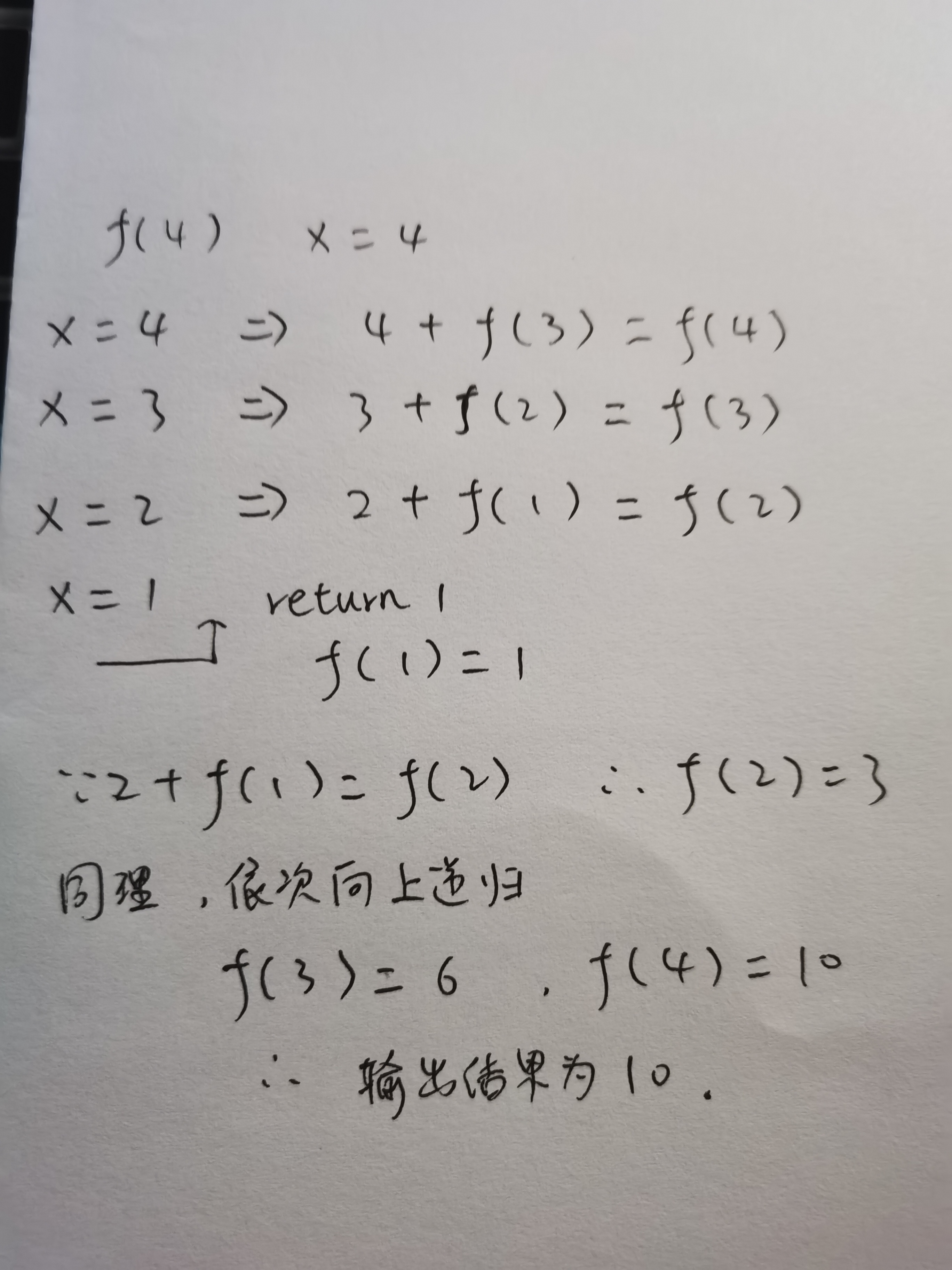# 一、函数的定义

## （一）def关键字定义函数

def后跟函数名和圆括号`()`，括号内是参数（参数也可以为空，此时则称该函数为`无参函数`），且函数内容以冒号`:`来表示这个函数的起始。

``````def 函数名 (参数或参数列表):
函数体
[return 语句]
``````

# 二、函数的调用

``````def input_number():
print("这是一个无参函数。")
def number_max(x, y, z):
print(x, y, z)
print("\t")

input_number()
number_max(3, 0, 1)

``````# 三、函数的参数传递

## （一）必需参数

``````def div(num1, num2, num3):
print(num3 + num1 - num2)
div(3,2,9)
def content_input(content):
print(content)

content_input("内容。。。")

``````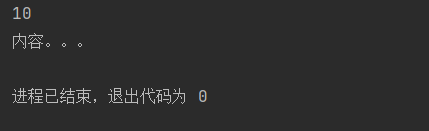## （二）关键字参数

``````def info(name, sex, age):
print("名字: ", name)
print("性别: ", sex)
print("年龄: ", age)

info(name="xiaoming", sex="男", age=18)

``````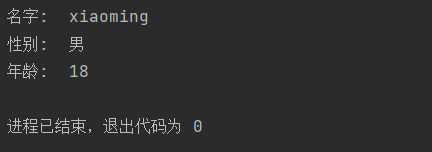## （三）默认参数

``````def info(name, sex, age, garde=1):
print("名字: ", name)
print("性别: ", sex)
print("年龄: ", age)
print("年级: ", garde)

info(name="xiaoming", sex="男", age=18)

``````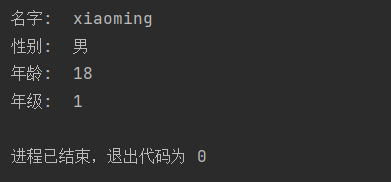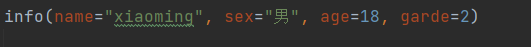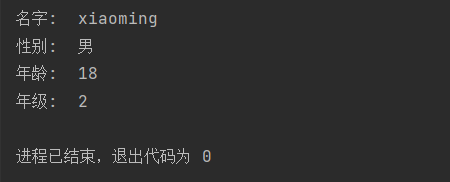## 1、元组形式导入（*）

``````def f(the_one, *the_else):
print(the_one)
print(the_else)

f(2, 3, "s", "t", 0, "c")

``````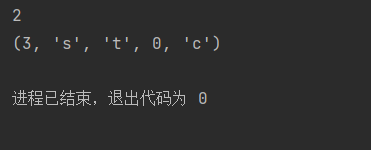## 2、字典形式导入（**）

``````def f(the_one, **the_else):
print(the_one)
print(the_else)

f(2, a=0, b=0.1, c=0.2)

``````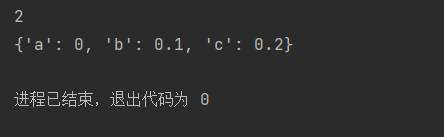# 四、匿名函数

``````number_sum = lambda a, b, h: (a + b) * h * 0.5
print(number_sum(2, 3, 1.5))

``````# 五、递归函数

`递归函数`，它可直接或间接地调用函数自身。其中函数递归时，要有递归公式和边界条件，递归公式用于分解规模较大的问题为规模较小的问题，边界条件用于终止递归函数。

``````def f(x):
if x == 1:
return 1
else:
return x + f(x - 1)

print(f(4))

``````